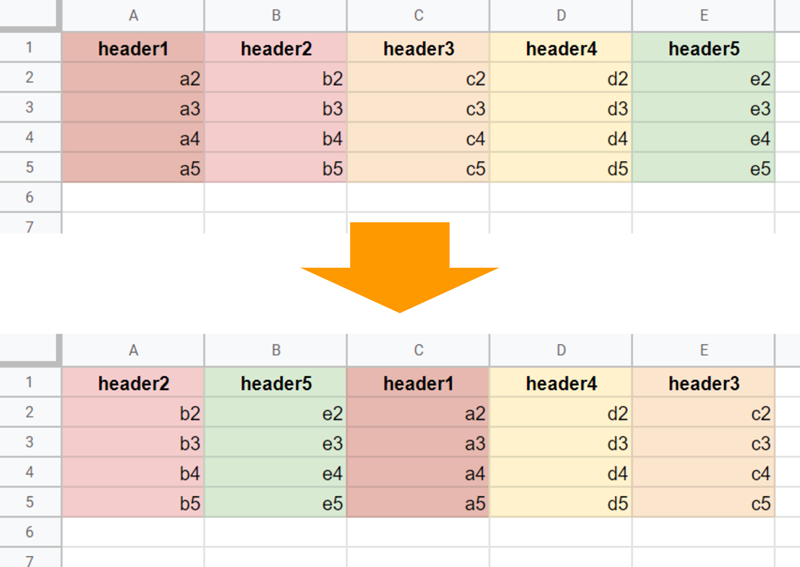Gists## Sample script

function myFunction() {

const obj = sheet
.getRange(1, 1, 1, sheet.getLastColumn())
.getValues()
.reduce(
(ar, h, i) => [...ar, { from: i + 1, to: finalOrder.indexOf(h) + 1 }],
[]
)
.sort((a, b) => (a.to > b.to ? 1 : -1));

for (let i = 0; i < obj.length; i++) {
if (obj[i].from != obj[i].to) {
sheet.moveColumns(sheet.getRange(1, obj[i].from), obj[i].to);
obj.forEach((e, j) => {
if (e.from < obj[i].from) obj[j].from += 1;
});
}
}
}

• When moveColumns(columnSpec, destinationIndex) is used, the indexes of columns are changed every run of moveColumns(columnSpec, destinationIndex). So, it is required to update the current column position. Please be careful about this.